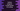# Python program to pad zeroes to a string## Python program to pad zeros to a string :

In this python programming tutorial, we will learn how to pad extra zeros to a string. For example, if the string is 2, and if we pad three zeros to this string, it will become 0002. Similarly, we can pad zeros to any type of string like 00Hello or 000world. We can pad any number of zeros to a string.

## Using zfill() to a string :

Python comes with a lot of useful utility methods. Even for padding extra zeros to a string, we can use zfill() method. The syntax of zfill() is as below :

```````str.zfill(width)
```````

width is the final width of the string. This width sized string will be created after zeros are padded to the string. This method will return the final string.

For example, if the value of width is 10 and the length of the string str is 5,_ zfill()_ will return a string with five zeros padded to the left.

Again, if the value of width is 5 and the length of the string is 10, zfill() will not add any zeros to the string as the length is already greater than the width. It will return the same string without doing any modification.

### Python example program :

Let’s try to implement zfill() method in python:

### Explanation :

The commented numbers in the above program denote the step number below :

1. Ask the user to enter a string. Read and store it in inputstr_ variable. We are using the ‘input()’ method to read the user input. This method reads the user input value as a string.
2. Ask the user to enter the count of zeros to insert to the left of the string.
3. Find the total count, i.e. the final size of the string including the zeros.
4. Print the final zero-padded string to the user. The zfill method takes the total count of letters as an argument. For example, if you want to print 002 for string 2, then you need to pass 3 as the argument to zfill().

### Sample Output :

We can also use_ zfill()_ with positive or negative signed number strings. Actually, zfill will see the value as a string, not as a number. If the value is_ “+120”, _zfill() will consider it as a string of length 4. If the width is 6, it will add two zeros to the start of the string. Note that the zeros will be added after the sign. So, zfill(6) for “+120” will result “+00120”, not “00+120”.

If the string has multiple signs, it will consider only the first one.

### Use cases :

zfill() method is used to pad zeroes to the start of a string. We can use_ zfill()_ if we want to return a constant length string from a function. Before returning any value from the function, use zfill() to adjust its length.

### Conclusion :

We have seen how to pad zeros to the left of a string using python. zfill() method is really helpful for padding zeros to a string or for converting a string to a predefined length. Try the examples above and drop a comment below if you have any query.